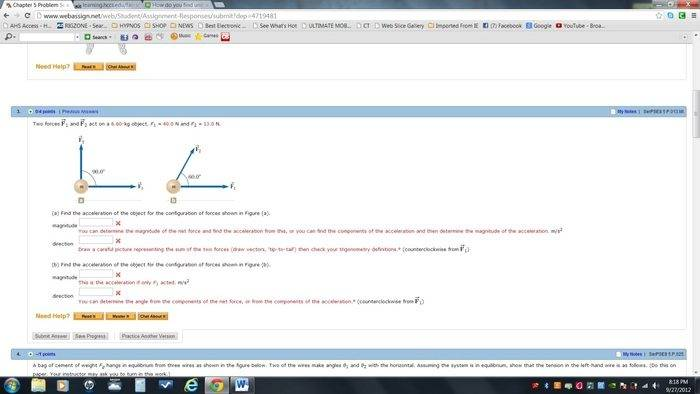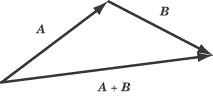# Two forces acting upon an object.

AryRezvani

## Homework Statement## Homework Equations

I assume, it has to do with components and forces so Fx = F Cos θ, and vice versa for the y component.

## The Attempt at a Solution

Well, initially, I tried to add them using unit vectors and got 40.0i + 13.0j for ƩF

Solved for vector acceleration using Ʃf = ma, and got 6.06i + 1.97j m/s^2.

a = ? θ = ?

Homework Helper
Draw the vectors head-to-tail ... then it will be clear.

AryRezvani
Draw the vectors head-to-tail ... then it will be clear.

Sorry, I'm still a little lost.

I connected the arrow heads together and got a triangle with 13.0 N acting upwards and 40.0 N acting to the right.

Change these all to acceleration using f = ma, and got 6.06 m/s2 to the right and 1.9696 m/s2 upwards.

Used pythag to solve for the acceleration vector and got 6.372 m/s2

For angle, I used Tan-1 (ay/ax) and it came out wrong.

AryRezvani
Okay, went ahead and redid it using a textbook method. Basically isolated the x and y components

fx = 40 N
fy = 13 N

Solve for acceleration using vector fx = max, and do the same for the fy

ax = 6.0606
ay = 1.9696

Tan-1 (ay/ax) which is equal to 10.39 degrees.

Plug that in, and it's incorrect. Any suggestions?

martix
What Simon was referring to is called Vector Addition. You could look that up and its corresponding algebraic expression.

AryRezvani
What Simon was referring to is called Vector Addition. You could look that up and its corresponding algebraic expression.

Doesn't that only solve the magnitude? So far, I've found the magnitude for both equations. I just don't understand why my angle keeps coming out wrong.

martix
A vector is an object that has 2 components(magnutude and direction), it would then be logical that adding 2 of those would again result in another object with those same components(i.e. again both magnitute and direction, not just one or the other).

Using the tip-to-tail method as suggested would present a very clear geometric way of deriving the answer - you'd get a triangle for which you'd need to find one angle and one side, by knowing everything else.

AryRezvani
A vector is an object that has 2 components(magnutude and direction), it would then be logical that adding 2 of those would again result in another object with those same components(i.e. again both magnitute and direction, not just one or the other).

Using the tip-to-tail method as suggested would present a very clear geometric way of deriving the answer - you'd get a triangle for which you'd need to find one angle and one side, by knowing everything else.

Okay, thanks you both for taking the time to respondI'll go ahead, and give it a go:

So we have f1 and f2

f1 = 40.0 Newtons
f2 = 13.0 Newtons

Mass of the object being 6.60 kg.

So, in regards to the suggested tip-to-tail method, I'll begin by drawing out a triangle on my paper.

One arrow points to the right with a magnitude of 40 Newtons. Attached to the tip is the tail of another arrow going directly upwards with a magnitude of 13.0 Newtons. That makes the angle at the point of intersection 90°.

So I'm going to stop here and ask a quick question. Is it necessary to use pythag to find the missing side?

I'm sorry if you feel like I'm over complicating it. I really want to understand this concept as a whole.

Homework Helper
So, in regards to the suggested tip-to-tail method, I'll begin by drawing out a triangle on my paper.

One arrow points to the right with a magnitude of 40 Newtons. Attached to the tip is the tail of another arrow going directly upwards with a magnitude of 13.0 Newtons. That makes the angle at the point of intersection 90°.

So I'm going to stop here and ask a quick question. Is it necessary to use pythag to find the missing side?

The resultant vector is an arrow drawn from the tail of the first vector to the head of the second one. This makes the hypotenuse of a right-angled triangle (in this case).

The length of the hypotenuse is the magnitude of the resultant so you will need pythagoras here.
The angle between the hypotenuse and the first vector is the angle of the resultant.

Read back through your notes and coursework: there will be several examples. Also look it up online - there are lots of tutorials like this one:

In general you won't have a right-angled triangle ... in general, geometric vector addition looks like this:Last edited:
martix
That'd be the easiest way for this particular case, yes. Also makes it easier to find the angle.
For the other example you'd have to get a little bit more into trig.